# OECD Non-Financial Corporations Debt to Surplus Ratio Analysis 5 - arranging data frame and make a bar chart using R.UnsplashPierre Lemosが撮影した写真

Thsi post is following of the above post.

In this post I will arrange data frame with arrange() function of dplyr packkage.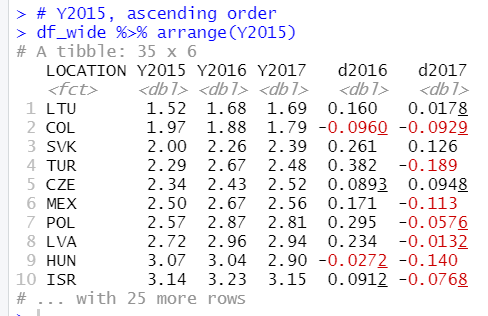Let's make a graph with ggplot2 packages.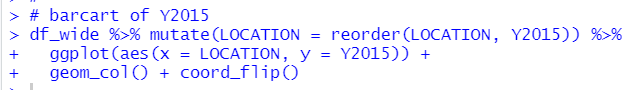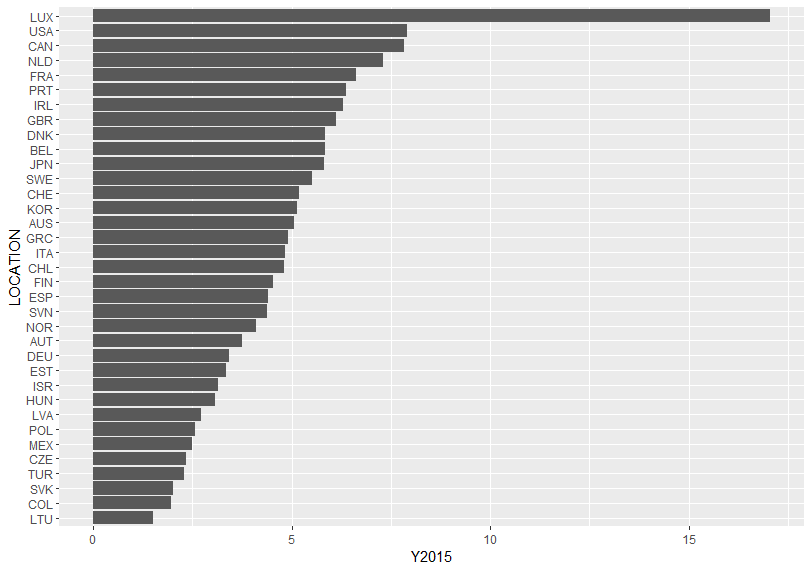LUX has the highest debt to surplus ratio in 2015. LTU has the lowest debt to surplus ratio in 2015.

Let's see Y2016.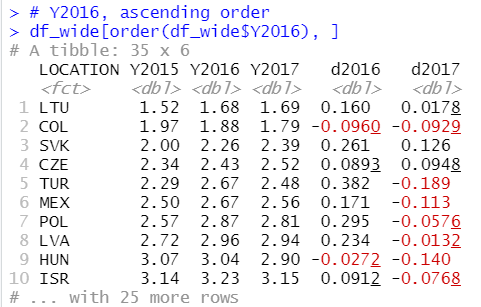This time, I use order() function instead of arrange() funtion.

Let's see a bar chart.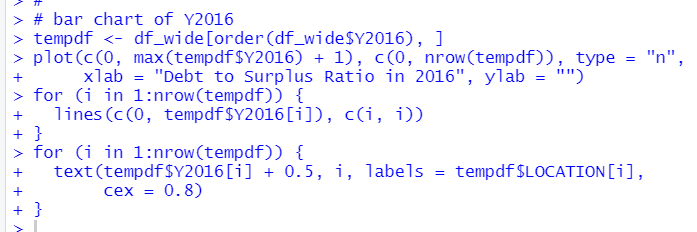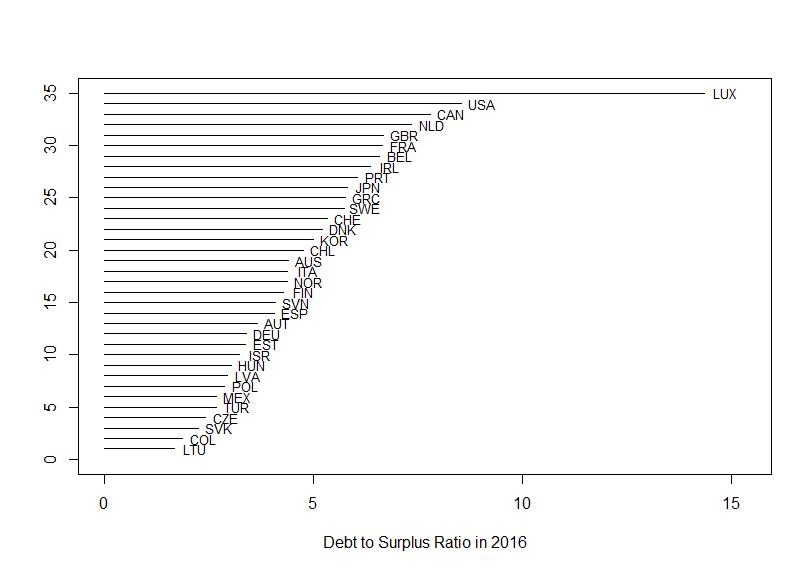This time I used plot() function, lines() function and text() function instated of using ggplot2 package.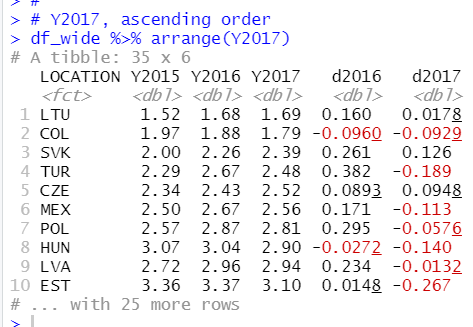Let's make a bar chart.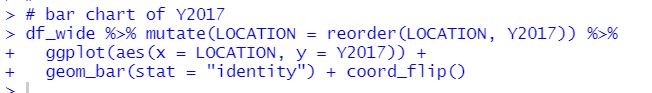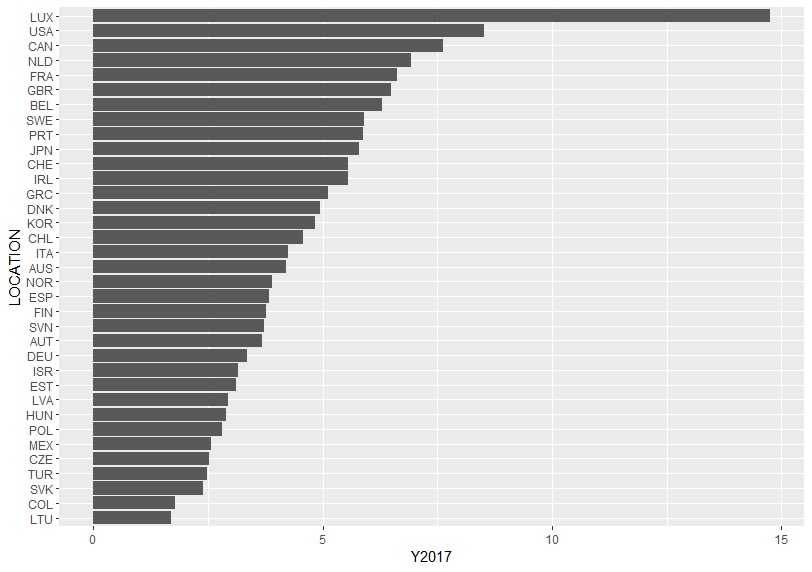This time I used geom_bar(stat = "identity") instead of geom_col() function.

LTU has the lowest and LUX has the higest.

Let's see d2016, it is change from Y2015 to Y2017.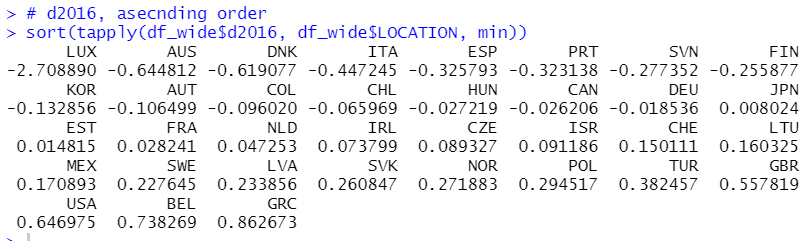This time I use tapply() function and sort() function. LUX has the smallest(negative) change and GRC has the largest(positive) change.

Let's make a bar chart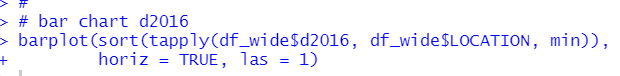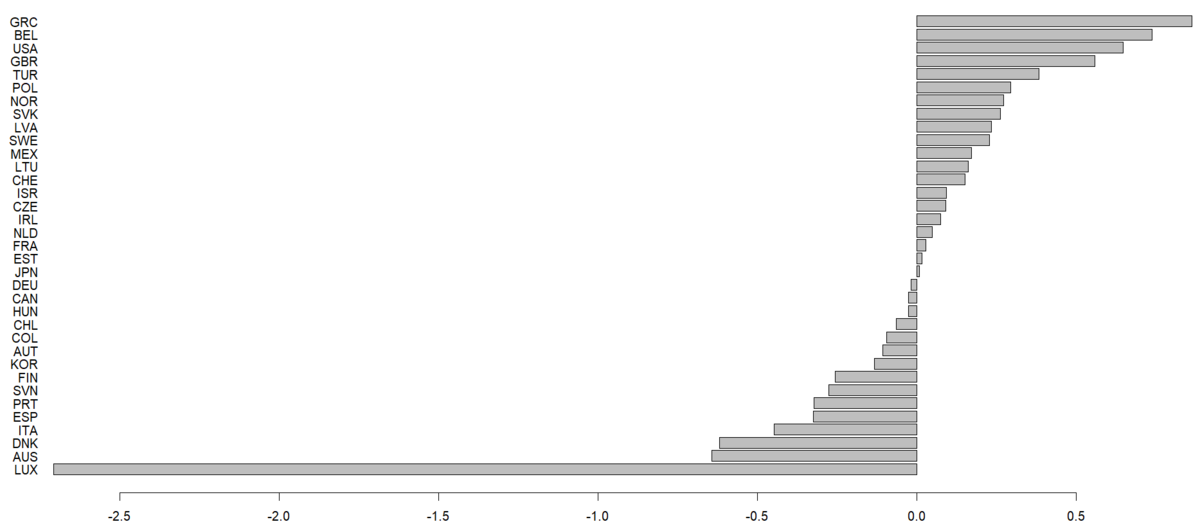This time I used barplot() function.

Let's see d2017, it is change from Y2016 to Y2017.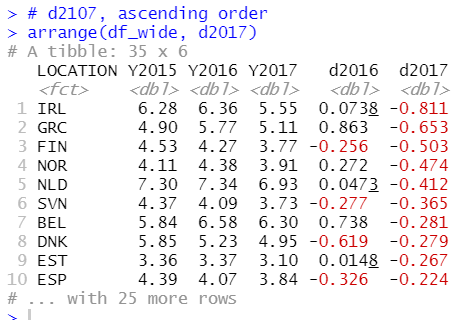Let's make a bar chart.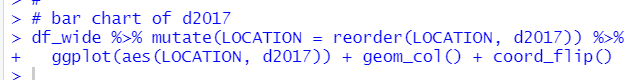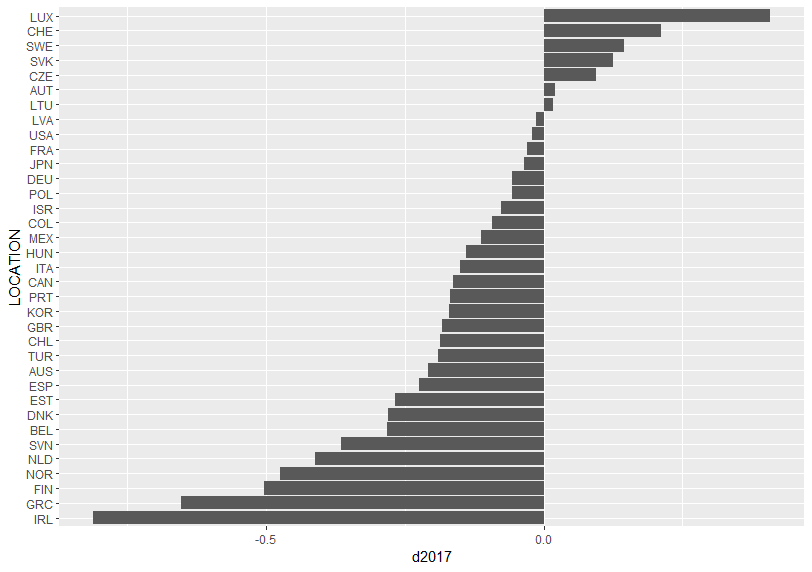LUX has the highest(positive) change and IRL has the lowest(negative) change.

That's it. Thank you!

Next post is